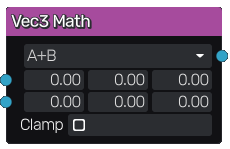# Vec3 Math node¶

The Vec3 Math node performs various vector math operations between its inputs.## Inputs¶

The Vec3 Math node accepts two color inputs. Those inputs are optional, and when left unconnected, the corresponding parameter value is used

## Outputs¶

The Vec3 Math node generates a single color texture that contains the result of the vector math operation.

## Parameters¶

The Vec3 Math node accepts the following parameters:

• the operation to be performed

• default values to be used in place of the inputs when left unconnected

• a boolean that specifies if the result must be clamped between 0 and 1